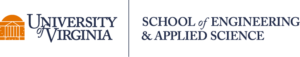### How do I use subgoals?

Subgoal labelled worked examples and practice problems are effective when students are beginning to learn a new concept. You can present the subgoal labels first (as pre-teaching, see Figure 1) before explaining the concept, or immediately after explaining the concept. You can then present one or more subgoal labelled worked examples (see Figure 2) to show how the subgoal labels are applied with the concept. ​

Figure 1. Subgoal labels for selection statements

Figure 2. Sample worked example

Following the explanation of the worked example(s), students should have the opportunity to practice using the subgoal labels to solve both similar and novel problems – practice problems (see Figure 3). At this point it is helpful if students have a reference sheet with the subgoals (see Figure 1) on it while working through the practice problems.

Figure 3. Sample practice problem

Subgoal labels can also be used for formative assessment in various ways:

1. Present a problem with the subgoal labels only and have students write the code for one or more subgoal labels
2. Present a problem with its solution and have students identify which parts of the solution belong to which subgoal label
3. Use Parsons problems (with or without subgoal labels) as a lower cognitive load means to demonstrate solution knowledge
4. Have students compare solutions to similar problems and compare the similarity and differences for the code belonging to each subgoal label.
Using the eBook We have developed an eBook containing worked examples, practice problems, and formative assessments for use in an introductory programming course taught in Java. The chapters are:
• Expression Statements
• Object Usage
• Evaluating Selection Statements
• Writing Selection Statements
• Evaluating Loops
• Writing Loops
• Writing Methods
• Writing Classes
• Evaluate Arrays
Each chapter contains the subgoal labels associated with the concept and one or more subgoal labeled worked examples – presented as text and a video explanation of the worked example. This is followed by auto-graded practice problems (multiple choice, fill-in-the-blank, Parsons problems). Formative assessments are built-in at appropriate times.

Note that the eBook contents are not necessarily meant to be done in a linear order, instead we have grouped them by subgoals. For example, evaluating boolean expressions, evaluating expressions with pre and post increment/decrement operators, and evaluating expressions with method calls are within the Expressions chapter because they all utilize the expressions subgoal labels. However, these likely wouldn’t be introduced when general arithmetic expressions are introduced, they usually occur with other topics (i.e., evaluating boolean expressions before selection statements, evaluating method calls with object usage). The book is designed so that you can assign the relevant topics as needed during the semester with minimal pre-requisite knowledge from other topics.

Ideas for how you might incorporate the worked examples and practice problems into your course:

• We highly encourage you to either present the worked examples in class OR present the video of the worked examples in class. When we assigned students to watch the videos before doing the practice problems, many students completely skip watching the videos or looking over the worked examples before attempting the problems. This can reduce the effectiveness of the intervention.
• Practice problems can be done during a lab period or assigned as homework.
• Grade the practice problems for completion / attempt only. Students learn from receiving the formative feedback from the auto-graded problems.
• You may use the quizzes for correctness grades.